What is ADC and How does it work?

The ADC is basically a system that converts an analog signal, such as sound, temperature, wind-speed, orientation, angular-motion, and many more into binary bit streams. The process of converting this information from one form into another can be seen in many aspects of our daily lives – for example: when we convert what we hear on the radio (analog) into music files stored away on your computer’s hard drive and then back again so you can listen via headphones!.

Another Example is Phone camera converts the image sensor values into digital image file. Same Process is reversed when an image is displayed back on a screen.

A Complex example of ADC is used in Car-Engine when hundreds of Engine parameters are measured via Analog sensors and converted into binary data and sent to the Engine Control Unit for processing.

1. Analog Sensitivity of ADC Pin How small voltage signal/value can be detected on the MCU -ADC Pin.
2. Converting Analog value into 8-12bits binary Data.
3. Sample Rate.

ADC is specified as 8-bit, 10-bit, 12-bit. Any Analog data needs to be converted into bit streams. Say 0 to 3.3V needs to be measured in 8 bits.

8 bits of ADC data = Maps Vin in 255 values.[0V -3.3/3.5/5V]

So, 1-bit value = 3.3/255 = 0.01294 v = 12.9 mV. This means an 8-bit ADC is sensitive enough to measure 12.9mV value at ADC.

How to interpret the value.

Say A temperature Sensor Can read values between 0-100C. The same value is translated into 0-3.3V range.

A 8-bit ADC can quantize   100/255 =0.392 Degree Centigrade as a single bit.

ADC is also defined by the sampling rate. How frequently you can read/sense the pin data. It can be as low as 100 Hz and as high as 40Mhz. To

Can you Measure Negative voltage on ADC?

ADCs are designed to detect Voltage range between 0(GND) and Vcc. Any Negative Voltage could damage your ADC module or the complete MCU. But there is a way to measure the Negative Voltage. For example, if you want to sample a sine wave [-2v to +2v] then You should put a voltage- divider Circuit before your MCU-ADC Pin. <<Add a Voltage Divider link here.>>

What is DAC and How does it work?

Digital to Analog Converters works by converting the digital value into analog. The range of Analog value is defined by  Digital-Val x  (Vref/2^n)

Where Vref is the input voltage at the power supply in 3.3V,3.6V or 5V.

N: represents the number of SAR Bits.

Why do we need ADC and DAC?

We need ADC to convert an analog Data from sensors into a Digital input which can be then used by Microcontrollers to Process the Data. Since MPUs understand only binary data-formats.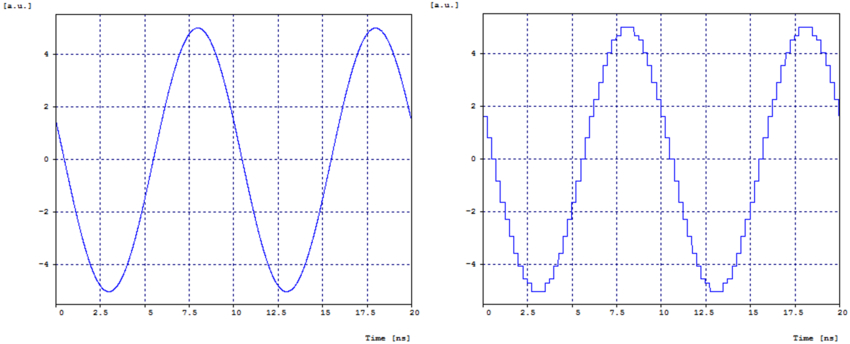Vice-versa When Microcontroller wants to send some Digital Output DAC converts Digital output to Analog voltage output which is understood by Analog Circuit.

PS: Now many Sensors with Analog inputs have an inbuilt ADC & DAC input which are calibrated for optimum performance and they interface via I2C/SPI.

Sensors and Device List using ADC/DAC

MPL3115A2 //add the exact model number

Humidity Sensor: AM2302/DHT22. //add the exact model number

Gyroscopes & Accelerometers use the

Voltage Divider Circuit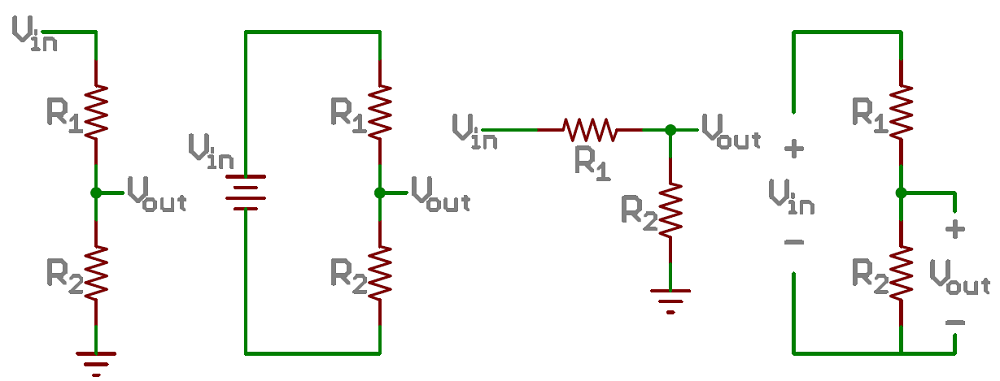Voltage Divider Circuit for DAC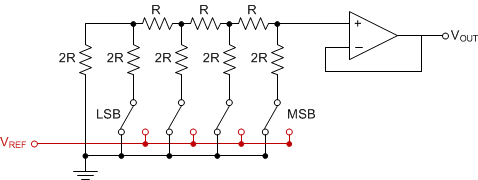ADC and DAC Module in MicroPython

The DAC is used to output analog values (a specific voltage) on pin X5 or pin X6. The voltage will be between 0 and 3.3V.

Example Code:

import math

from pyb import DAC

# create a buffer containing a sine-wave

buf = bytearray(100)

for i in range(len(buf)):

buf[i] = 128 + int(127 * math.sin(2 * math.pi * i / len(buf)))

# output the sine-wave at 400Hz

dac = DAC(1)

dac.write_timed(buf, 400 * len(buf), mode=DAC.CIRCULAR)

The above code will output a sine wave

How to Debug ADC and DAC

ADC can be sensitive to noise leading to large discrepancies in ADC readings. To minimize noise, users may connect a 0.1 µF capacitor to the ADC input pad in use. Multisampling may also be used to further mitigate the effects of noise.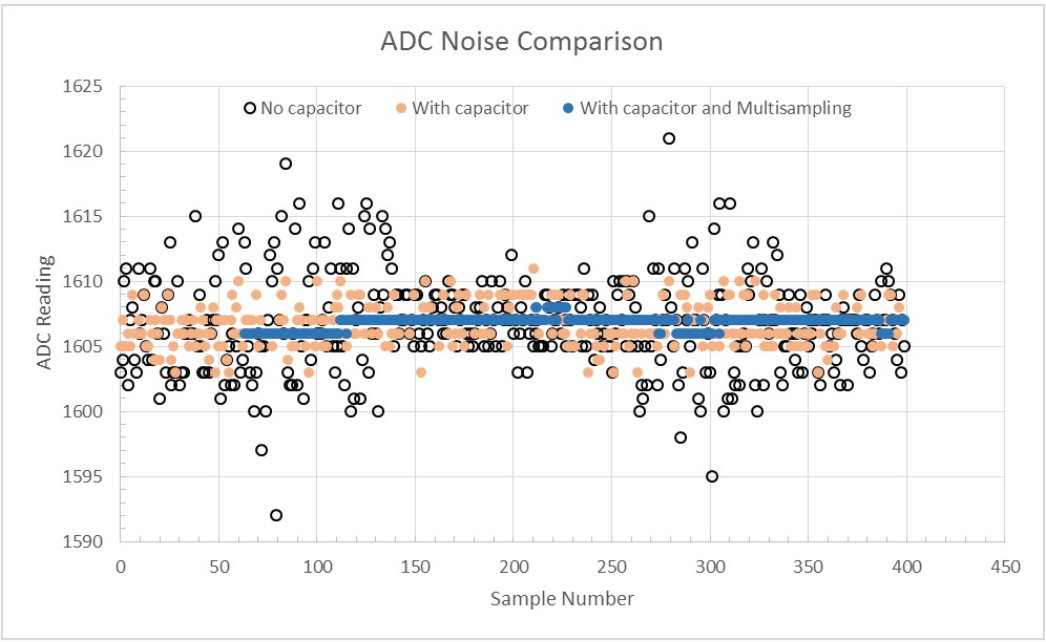Calibration: It is a good practice to add a 0.1uf Capacitor before the ADC pin. This helps in filtering out the noise and any static dv voltage built-up

Concurrent reading Sampling Multiple values from ADC pins and evaluating  the most concurrent reading and doing an average is a good practice. Especially When the reading values are in low range.

A median Algo is a good start to read the values.

Copy/Write Error:

Many times, 10 bit or 12-bit ADC  value is read into the u16 datatype. It is good practice to reset the value to 0 after the operation.

Sample over Nyquist rate : If you are doing signal reconstruction and doing some Signal Processing like FFT(MicroPython) . you can refer to this library.

https://github.com/peterhinch/micropython-fourier

ESP32 ADC DAC Programing and Pin Out

Sampling Rate = 6KHz = 6000 samples/sec

Pinout-Diagram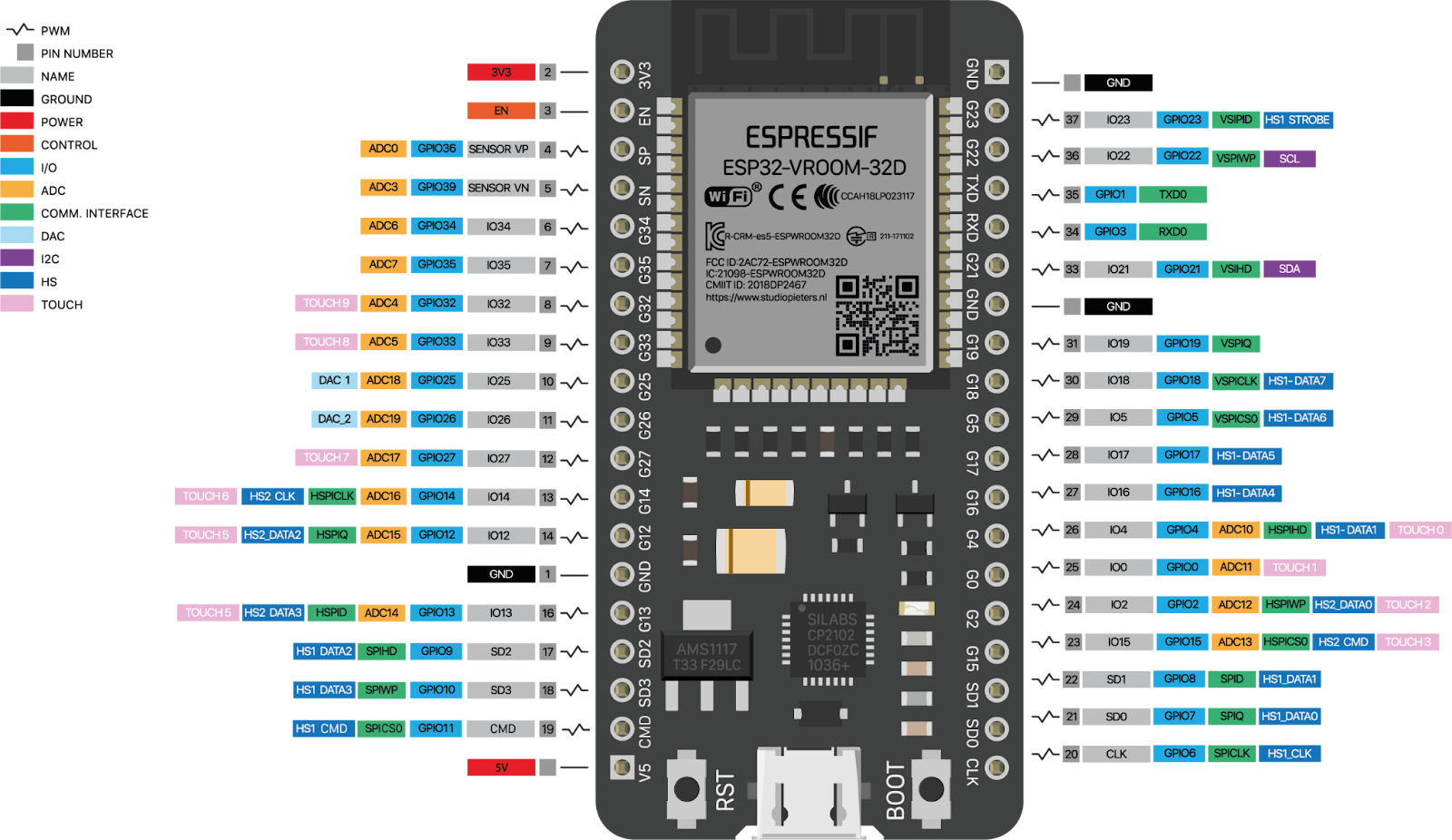ESP32 has 2 ADC blocks built into the silicon.

GPIO32

GPIO33

GPIO34

GPIO35

GPIO36

GPIO37

GPIO38

GPIO39

EACH ADC IS 12-bit ADC by default, can be configured to 10-bit and 11-bit

• Some of the ADC2 pins are used as strapping pins (GPIO 0, 2, 15) thus cannot be used freely. Such is the case in the following official Development Kits:
• ESP32 DevKitC: GPIO 0 cannot be used due to external auto program circuits.
• ESP-WROVER-KIT: GPIO 0, 2, 4 and 15 cannot be used due to external connections for different purposes.
• Since the ADC2 module is also used by the Wi-Fi, only one of them could get the preemption when using together, which means the adc2_get_raw() may get blocked until Wi-Fi stops, and vice versa.

Sample Code

from time import sleep
import utime
sample_rate=100 #max value 6000

while True:
adc_value = 0 #re-initialize val to zero
utime.sleep(1/sample_rate)

Sample OutputArduino ADC and DAC Programming and Pinout.

Sampling rate = 9.6KSPS(9600)

The ADC clock of Atmega328P is 16 MHz divided by a ‘prescale factor’. The prescale is set by default to 128 which leads to 16MHz/128 = 125 MHz ADC clock. Since a single conversion takes 13 ADC clocks, the default sampling rate is ~ 9600 Hz.

This the default Sample rate unless the interrupts are disabled and the prescale factor is reduced.

Pinout-Diagram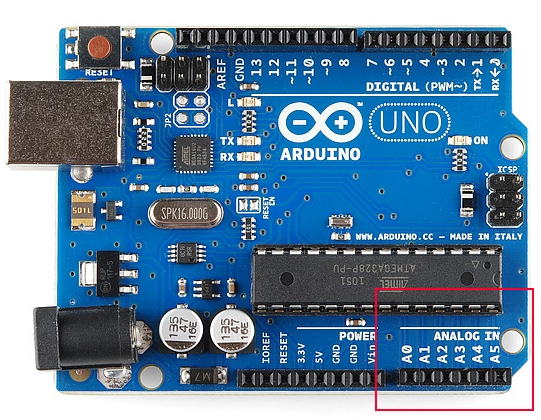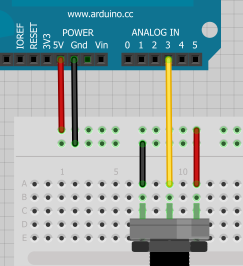Sample Code

int analogPin = A3; // potentiometer wiper (middle terminal) connected to analog pin 3
// outside leads to ground and +5V
int val = 0;  // variable to store the value read
void setup() {
Serial.begin(9600);           //  setup serial
}
void loop() {
Serial.println(val);          // debug value
}

Sample OutputRaspberry Pi PICO ADC and DAC Programming and Pinout.

Sample Rate = 500kSPS

Number of ADC Channels = 4

Pinout-Diagram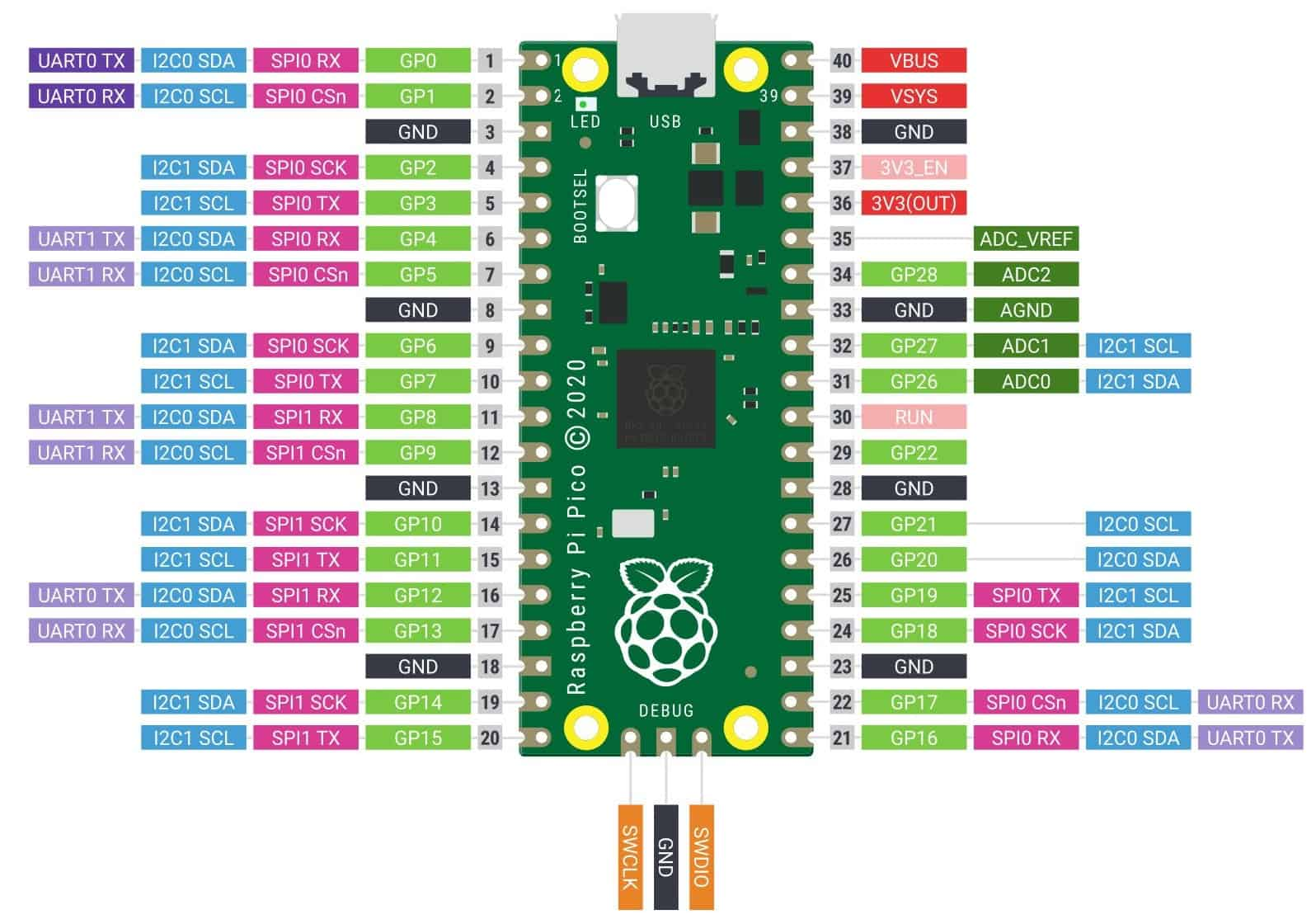Sample Code

from time import sleep
import utime

sample_rate=100 #max value 6000

while True:
adc_value = 0 # resetting the value to zero
utime.sleep(1/sample_rate)

Sample Output